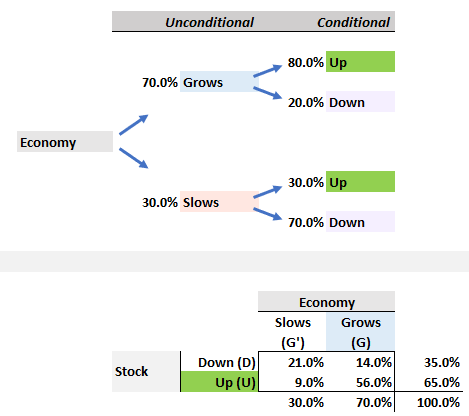# Bayes Theory

#### Eustice_Langham

##### Active Member
Hi David, I have a question concerning an old video on YT concerning the Bayes Theory.
, my question concerns how you have arrived at the values in the formula, specifically the values in the denominator of P(U).
In the formula, you have:
P(G/U) = P(U/G) * P(G) / P(U)
= (.8)*(.7) / ((.8)*(.7)) + ((.3)*(.3))

My question is why is P(U), in the denominator = .3 * .3 and not equal to .3?

The only up .3 that I can see is when the economy slows ie .3 the stock only has a .3 chance of going up.

Thanks

#### David Harper CFA FRM

##### David Harper CFA FRM
Staff member
Subscriber
Hi @Eustice_Langham We're applying Bayes to solve for P(G|U) = P(U,G)/P(U) = [P(U|G) * P(G)] / P(U). The denominator is the unconditional P(U) which is the sum of two joint probabilities: P(U) = P(S,U) + P(G,U) = (70%*80%) + (30%*30%). For me, it's almost always helpful to translate the tree into a probability matrix (see lower panel below). Given the probability matrix, P(G|U) is solving for 56.0%/65%. Let me know if that still doesn't make sense? Thanks!#### Eustice_Langham

##### Active Member
Thanks David, it always helps to visualise the problem and this is where the tree and the matrix comes in handy. What I wasn't understanding was that the denominator is considered as the unconditional probability so it now makes sense to calculate the denominator the way it was, whereas Bayes is about conditional probability. Thanks again.